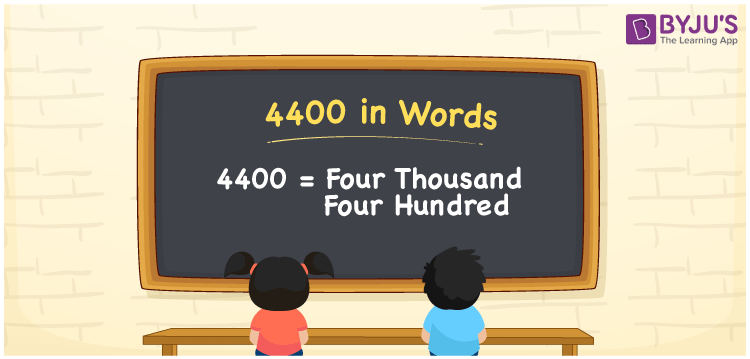# 4400 in Words

4400 in words can be written as Four Thousand Four hundred. For example if you buy a dress for Rs. 4400, then you can say that “I bought a dress for Four Thousand Four hundred Rupees”. The English alphabet is made use of to write 4400 in words. The step wise explanation given for writing numbers in words provides a strong conceptual base among the students. In English, 4400 can be read as “Four Thousand Four hundred”.

 4400 in words Four Thousand Four hundred Four Thousand Four hundred in Numbers 4400

## 4400 in English Words## How to Write 4400 in Words?

In this section, you will learn more about the concept of writing 4400 in words. There are four digits in the number 4400. Below you will find the place value chart which shows the place value of all the four digits.

 Thousands Hundreds Tens Ones 4 4 0 0

The expanded form of the number 4400 is provided below:

4 x Thousand + 4 × Hundred + 0 × Ten + 0 × One

= 4 x 1000 + 4 × 100 + 0 × 10 + 0 × 1

= 4000 + 400

= 4400

= Four Thousand Four hundred

Therefore, 4400 in words is written as Four Thousand Four hundred.

4400 is a natural number that precedes 4401 and succeeds 4399.

4400 in words – Four Thousand Four hundred

Is 4400 an odd number? – No

Is 4400 an even number? – Yes

Is 4400 a perfect square number? – No

Is 4400 a perfect cube number? – No

Is 4400 a prime number? – No

Is 4400 a composite number? – Yes

## Frequently Asked Questions on 4400 in Words

Q1

### Write 4400 in words.

4400 in words is Four Thousand Four hundred.
Q2

### What is the value of 4500 minus 100?

The value of 4500 minus 100 is 4400. Therefore, 4400 in words is Four Thousand Four hundred.
Q3

### Is 4400 a perfect cube?

No, 4400 is not a perfect cube as it cannot be expressed as the product of three integers which are equal.